# 《Machine Learning in Action》—— 剖析支持向量机，单手狂撕线性SVM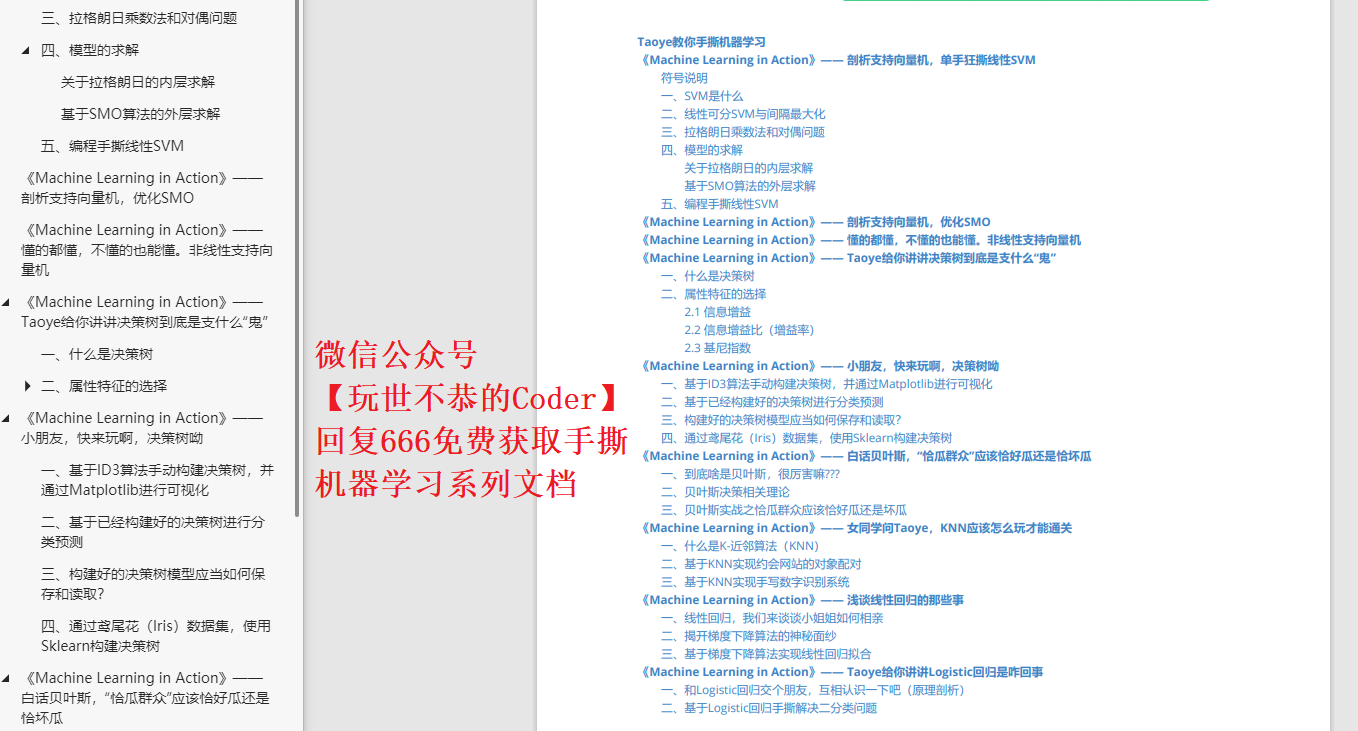• SVM内部的数学公式很多，但请不要未战先怯，犯下兵家大忌。无论是阅读该篇文章也好，学习其他相关SVM资源也罢，还请诸君耐心、认真且完整的看完。
• SVM的原理过程会涉及到很多的符号或记号，一定要梳理清楚他们所代表的含义。另外，推导过程中会存在很多的向量或矩阵，一定要明白其中shape，这一点可能在不同的资料中会有不一样的处理方式。
• 在阅读的同时，一定要拿出稿纸手动推演SVM的过程，尽可能明白整个过程的来龙去脉，有不明白的地方可以留言或查找其他相关资料来辅助自己的理解。
• 阅读一遍或许有不少知识不理解，但多阅读几遍相信一定会有不少收获

## 符号说明

x i x_i 表示单个样本，其中包含多个属性，显示为一个行向量
x ( i ) x^{(i)} 表示单个样本中的某个属性特征
y i y_i 表示单个样本所对应的标签（具体分类），为整数，非1即-1
w T w^T 表示的是权值行向量，其中 w = ( w 1 , w 2 , . . . , w m ) T w=(w_1,w_2,...,w_m)^T ，也是所需要训练的参数
b b 表示的是决策面中的 b b ，也是所需要训练的参数
γ ^ \hat{\gamma} 表示函数间隔，具体解释见下文
γ \gamma 表示几何间隔，具体解释见下文
α \alpha α = ( α 1 , . . . , α N ) \alpha=(\alpha_1,...,\alpha_N) 表示拉格朗日乘子
K i j K_{ij} 在这篇文章中表示线性核函数

## 一、SVM是什么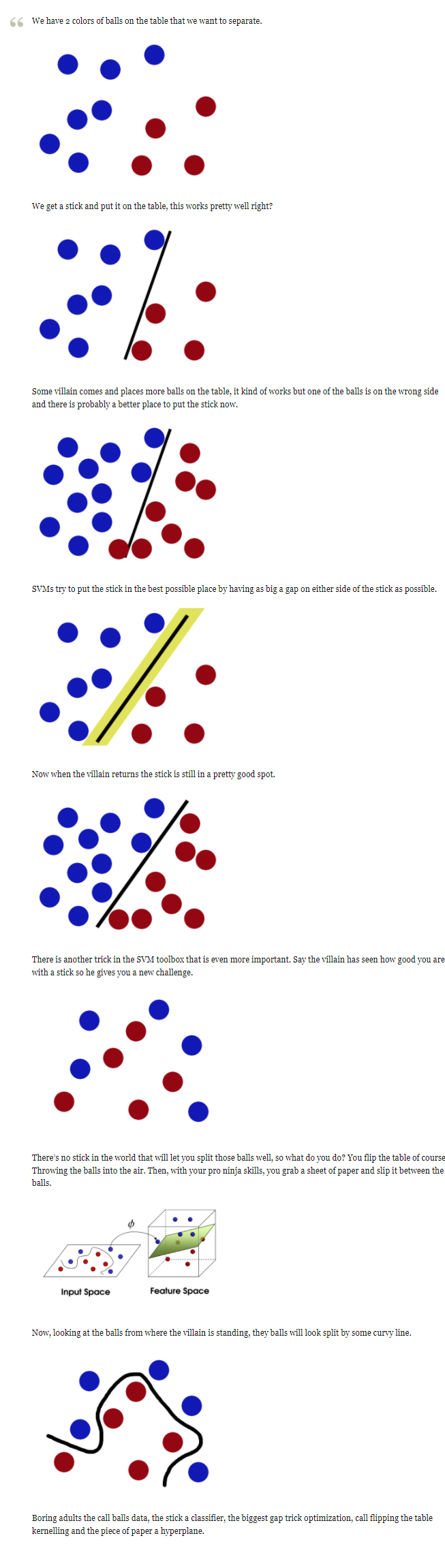## 二、线性可分SVM与间隔最大化

import numpy as np
import pylab as pl
from sklearn import svm

%matplotlib inline
print(np.__version__)

"""
Author: Taoye
微信公众号：玩世不恭的Coder
"""
if __name__ == "__main__":
# np.random.seed(100)        # 可自行设置随机种子每次随机产生相同的数据
X_data = np.concatenate((np.add(np.random.randn(20, 2), [3, 3]),
np.subtract(np.random.randn(20, 2), [3, 3])),
axis = 0)      # random随机生成数据，+ -3达到不同类别数据分隔的目的

Y_label = np.concatenate((np.zeros(), np.ones()), axis = 0)

svc = svm.SVC(kernel = "linear")
svc.fit(X_data, Y_label)

w = svc.coef_
ww = -w / w                # 获取权重w
xx = np.linspace(-6, 6)
yy = ww * xx - (svc.intercept_) / w   # intercept_获取结截距

b_down = svc.support_vectors_         # 得到对应的支持向量
yy_down = ww * xx + (b_down - ww * b_down)
b_up = svc.support_vectors_[-1]
yy_up = ww * xx + (b_up - ww * b_up)

pl.plot(xx, yy, "k-"); pl.plot(xx, yy_down, "k--"); pl.plot(xx, yy_up, "k--")
pl.scatter(X_data[:, 0], X_data[:, 1], c = Y_label, cmap = pl.cm.Paired)

pl.show()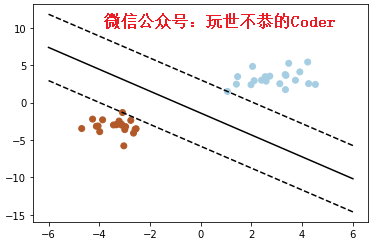A x + B y + C = 0 Ax+By+C=0

KaTeX parse error: Undefined control sequence: \ at position 151: …rix} \right) \̲ ̲ +b=0 \tag{2…

w = ( w 1 , w 2 ) T , x = ( x ( 1 ) , x ( 2 ) ) w=(w_1, w_2)^T, x=(x^{(1)}, x^{(2)}) ，则上述指向方程最终可以表示为:

w T x + b = 0 (2-2) w^Tx+b=0 \tag{2-2}

γ i ^ = y i ( w T x i + b ) (2-3) \hat{\gamma_i}=y_i(w^Tx_i + b) \tag{2-3}

γ ^ = m i n i = 1 , . . . , N γ i ^ (2-4) \hat{\gamma} = \mathop{min}\limits_{i=1,...,N}\hat{\gamma_i} \tag{2-4}

γ i = ∣ w T x i + b ∣ ∣ ∣ w ∣ ∣ (2-5) \gamma_i=\frac{|w^Tx_i + b|}{||w||} \tag{2-5}

γ i = y i w T x i + b ∣ ∣ w ∣ ∣ (2-6) \gamma_i=y_i\frac{w^Tx_i + b}{||w||} \tag{2-6}

γ = m i n i = 1 , . . . , N γ i (2-7) \gamma=\mathop{min}\limits_{i=1,...,N}\gamma_i \tag{2-7}

γ i = γ i ^ ∣ ∣ w ∣ ∣ ， 即 γ = γ ^ ∣ ∣ w ∣ ∣ (2-8) \gamma_i=\frac{\hat{\gamma_i}}{||w||}，即\gamma=\frac{\hat{\gamma}}{||w||} \tag{2-8}

γ i = y i w T x i + b ∣ ∣ w ∣ ∣ ≥ γ ， 即 y i ( w T x i + b ) ≥ γ ∣ ∣ w ∣ ∣ = γ ^ (2-9) \gamma_i=y_i\frac{w^Tx_i + b}{||w||}\geq\gamma ，即y_i(w^Tx_i + b)\geq\gamma||w||=\hat{\gamma} \tag{2-9}

m a x w , b γ ^ ∣ ∣ w ∣ ∣ s . t .   y i ( w T x i + b ) ≥ γ ^ ， i = 1 , 2 , 3 , . . . , N (2-10) \begin{aligned} & \mathop{max}\limits_{w,b} \quad \frac{\hat{\gamma}}{||w||} \tag{2-10} \\ & s.t. \quad\ y_i(w^Tx_i + b)\geq\hat{\gamma}，i=1,2,3,...,N \end{aligned}

m i n w , b 1 2 ∣ ∣ w ∣ ∣ 2 s . t .   y i ( w T x i + b ) − 1 ≥ 0 ， i = 1 , 2 , 3 , . . . , N (2-11) \begin{aligned} & \mathop{min}\limits_{w,b} \quad \frac{1}{2}||w||^2 \\ & s.t. \quad\ y_i(w^Tx_i + b)-1\geq0，i=1,2,3,...,N \tag{2-11} \end{aligned}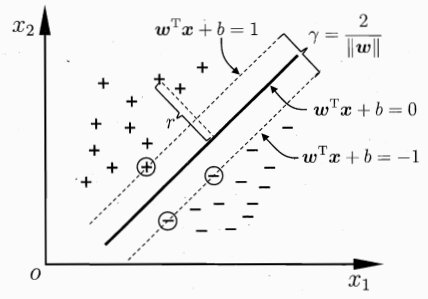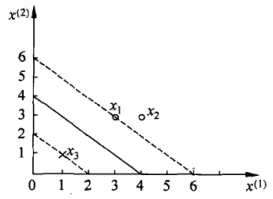m i n w , b 1 2 ( w 1 2 + w 2 2 ) s . t .    3 w 1 + 3 w 2 + b ≥ 1   4 w 1 + 3 w 2 + b ≥ 1 − w 1 − w 2 − b ≥ 1 \begin{aligned} & \mathop{min}\limits_{w,b} \quad \frac{1}{2}(w_1^2+w_2^2) \\ & s.t. \quad\ \ 3w_1+3w_2+b \geq1 \\ & \qquad \quad\ 4w_1+3w_2+b \geq1 \\ & \qquad \quad -w_1-w_2-b \geq1 \end{aligned}

1 2 x ( 1 ) + 1 2 x ( 2 ) − 2 = 0 \frac{1}{2}x^{(1)}+\frac{1}{2}x^{(2)}-2=0

## 三、拉格朗日乘数法和对偶问题

L ( x , y , λ ) = 4 x + x y 2 + y 2 + λ ( x 2 + y 2 − 1 ) L(x, y, \lambda)=4x+xy^2+y^2+\lambda(x^2+y^2-1)

{ L x ′ ( x , y , λ ) = 4 + y 2 + 2 λ x = 0 L y ′ ( x , y , λ ) = 2 x y + 2 y + 2 λ y = 0 L λ ′ ( x , y , λ ) = x 2 + y 2 − 1 = 0 \begin{cases} L_x^{'}(x,y,\lambda)=4+y^2+2\lambda x=0 \\ L_y^{'}(x,y,\lambda)=2xy+2y+2\lambda y=0 \\ L_\lambda^{'}(x,y,\lambda)=x^2+y^2-1=0\\ \end{cases}

m i n w , b 1 2 ∣ ∣ w ∣ ∣ 2 s . t .   y i ( w T x i + b ) − 1 ≥ 0 ， i = 1 , 2 , 3 , . . . , N (3-1) \begin{aligned} & \mathop{min}\limits_{w,b} \quad \frac{1}{2}||w||^2 \\ & s.t. \quad\ y_i(w^Tx_i + b)-1\geq0，i=1,2,3,...,N \tag{3-1} \end{aligned}

L ( w , b , α ) = 1 2 ∣ ∣ w ∣ ∣ 2 − ∑ i = 1 N α i y i ( w T x i + b ) + ∑ i = 1 N α i (3-2) L(w,b,\alpha)=\frac{1}{2}||w||^2-\sum^N_{i=1}\alpha_iy_i(w^Tx_i+b) + \sum^N_{i=1}\alpha_i \tag{3-2}

θ ( w , b ) = m a x α > 0   L ( w , b , α ) \theta(w,b)=\mathop{max}\limits_{\alpha>0} \ L(w,b,\alpha) ，原始目标函数即可转化为：

m i n w , b 1 2 ∣ ∣ w ∣ ∣ 2 = m i n w , b   m a x α L ( w , b , α ) = p ∗ (3-3) \mathop{min}\limits_{w,b} \frac{1}{2}||w||^2= \mathop{min}\limits_{w,b}\ \mathop{max}\limits_{\alpha}L(w,b,\alpha) =p^* \tag{3-3}

m a x α   m i n w , b L ( w , b , α ) = d ∗ (3-4) \mathop{max}\limits_{\alpha}\ \mathop{min}\limits_{w,b}L(w,b,\alpha) =d^* \tag{3-4}

• 疑问1：为什么前面我们令 θ ( w , b ) = m a x α > 0   L ( w , b , α ) \theta(w,b)=\mathop{max}\limits_{\alpha>0} \ L(w,b,\alpha) ，而不是其他的表达形式呢？

θ ( w , b ) = m a x α > 0   L ( w , b , α ) = m a x α > 0   [ 1 2 ∣ ∣ w ∣ ∣ 2 − ∑ i = 1 N α i y i ( w T x i + b ) + ∑ i = 1 N α i ] (3-5) \theta(w,b)=\mathop{max}\limits_{\alpha>0} \ L(w,b,\alpha)=\mathop{max}\limits_{\alpha>0} \ [\frac{1}{2}||w||^2-\sum^N_{i=1}\alpha_iy_i(w^Tx_i+b) + \sum^N_{i=1}\alpha_i \tag{3-5}]

∑ i = 1 N α i y i ( w T x i + b ) − ∑ i = 1 N α i ≥ 0 \sum^N_{i=1}\alpha_iy_i(w^Tx_i+b) - \sum^N_{i=1}\alpha_i \geq0

1 2 ∣ ∣ w ∣ ∣ 2 = > m a x α > 0   L ( w , b , α ) (3-6) \frac{1}{2}||w||^2 \quad => \quad \mathop{max}\limits_{\alpha>0} \ L(w,b,\alpha) \tag{3-6}

• 疑问2：为什么将原问题转化为对偶问题之后，在什么样的条件下，才会满足 d ∗ = p ∗ d^*= p^*

{ α i , i = 1 , 2 , 3 , . . . , N y i ( w T x i + b ) ≥ 0 , i = 1 , 2 , 3 , . . . , N α i ( y i ( w T x i + b ) − 1 ) = 0 , i = 1 , 2 , 3 , . . . , N (3-7) \begin{cases} \alpha_i, & i=1,2,3,...,N\\ y_i(w^Tx_i+b)\geq0, & i=1,2,3,...,N\\ \alpha_i(y_i(w^Tx_i+b)-1)=0, & i=1,2,3,...,N\\ \end{cases} \tag{3-7}

m a x α   m i n w , b [ 1 2 ∣ ∣ w ∣ ∣ 2 − ∑ i = 1 N α i y i ( w T x i + b ) + ∑ i = 1 N α i ] (3-8) \mathop{max}\limits_{\alpha}\ \mathop{min}\limits_{w,b}[\frac{1}{2}||w||^2-\sum^N_{i=1}\alpha_iy_i(w^Tx_i+b) + \sum^N_{i=1}\alpha_i] \tag{3-8}

1. 先求内层关于 w , b w,b 的最小值，此时我们应该将 α \alpha 视为常数
2. 再求外层关于 α \alpha 的最大值，由于经过了第一步关于 w , b w,b 的最小值，这两个参数已经被消掉了，所以第二步只会存在关于 α \alpha 的求解

## 四、模型的求解

### 关于拉格朗日的内层求解

m i n w , b L ( w , b ) = m i n w , b [ 1 2 ∣ ∣ w ∣ ∣ 2 − ∑ i = 1 N α i y i ( w T x i + b ) + ∑ i = 1 N α i ] (4-1) \mathop{min}\limits_{w,b}L(w,b)=\mathop{min}\limits_{w,b}[\frac{1}{2}||w||^2-\sum^N_{i=1}\alpha_iy_i(w^Tx_i+b) + \sum^N_{i=1}\alpha_i] \tag{4-1}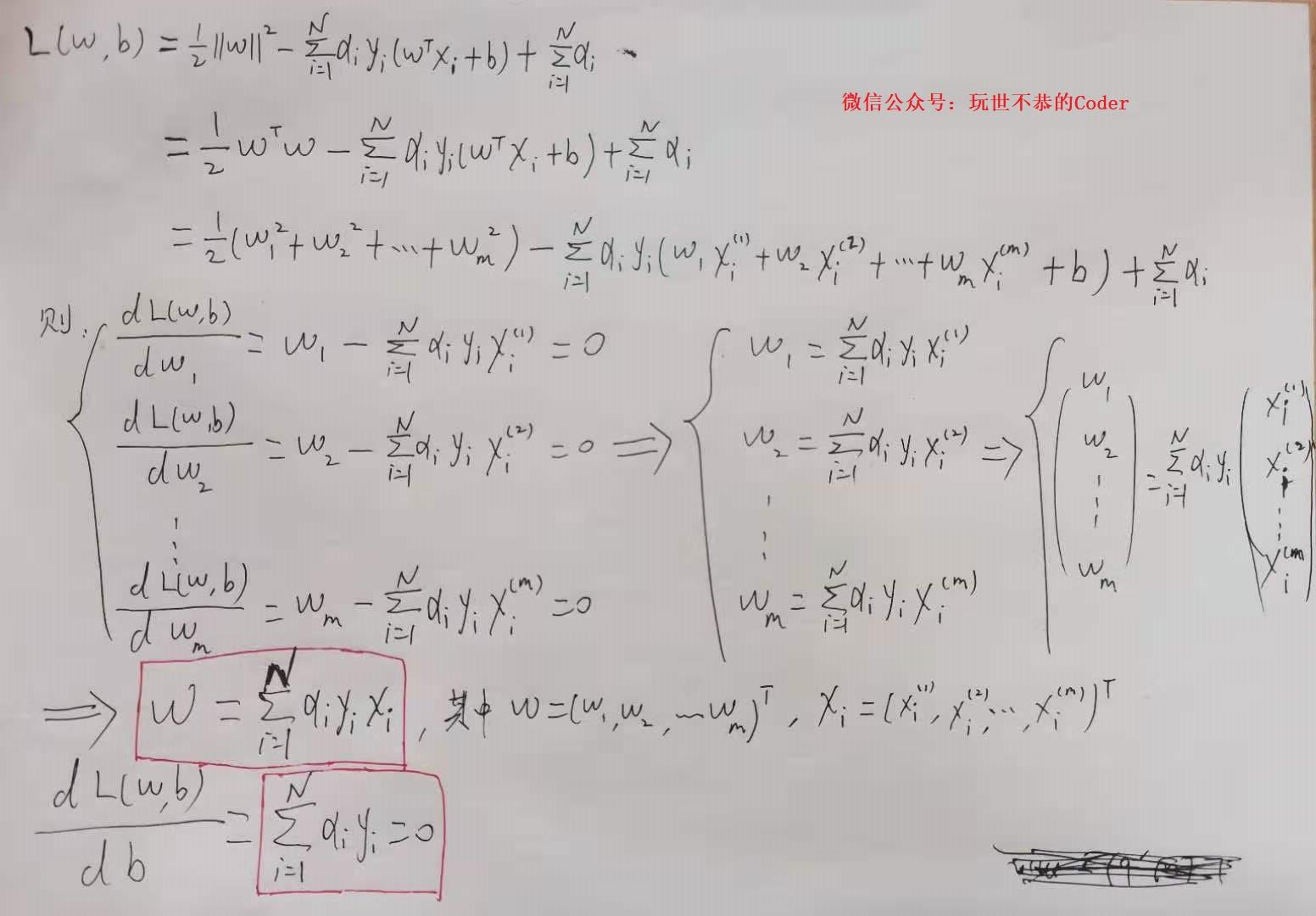w = ∑ i = 1 N α i y i x i 0 = ∑ i = 1 N α i y i (4-2) w=\sum_{i=1}^N\alpha_iy_ix_i \\ 0=\sum_{i=1}^N\alpha_iy_i \tag{4-2}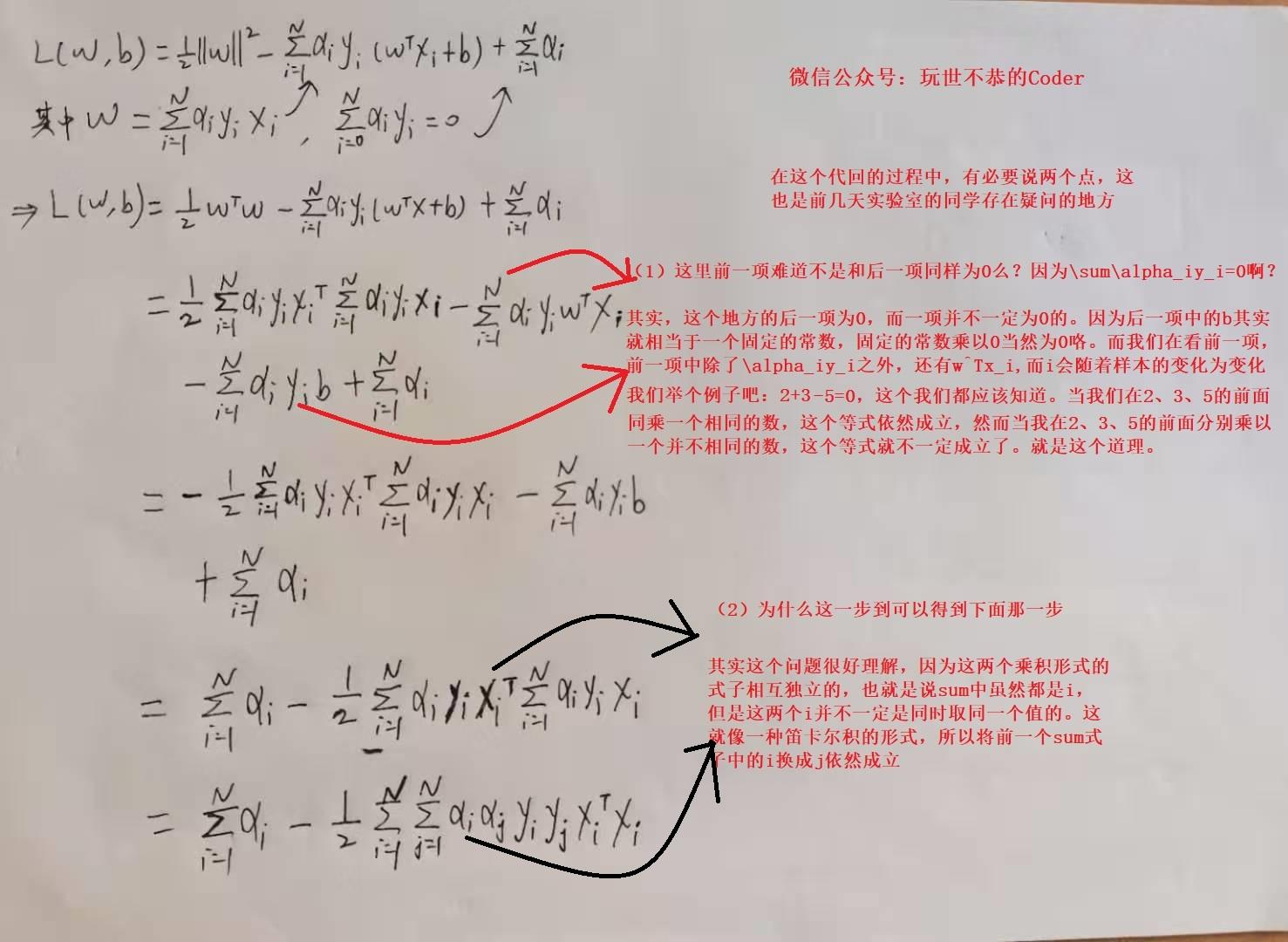• 疑问1：这里前一项难道不是和后一项同样为0么？因为不是说了 ∑ i = 1 N α i y i = 0 \sum_{i=1}^N\alpha_iy_i=0 啊？？？

• 疑问2：为什么这一步可以推导至下面那一步呢？？？

m i n w , b L ( w , b ) = ∑ i = 1 N α i − 1 2 ∑ i = 1 N ∑ j = 1 N α i α j y i y j x i T x i (4-3) \mathop{min}\limits_{w,b}L(w,b)=\sum_{i=1}^N\alpha_i-\frac{1}{2}\sum_{i=1}^N\sum_{j=1}^N\alpha_i\alpha_jy_iy_jx_i^Tx_i \tag{4-3}

m a x α   m i n w , b L ( w , b , α ) = m a x α   m i n w , b [ 1 2 ∣ ∣ w ∣ ∣ 2 − ∑ i = 1 N α i y i ( w T x i + b ) + ∑ i = 1 N α i ] = m a x α [ ∑ i = 1 N α i − 1 2 ∑ i = 1 N ∑ j = 1 N α i α j y i y j x i T x j ] = m i n α [ 1 2 ∑ i = 1 N ∑ j = 1 N α i α j y i y j x i T x j − ∑ i = 1 N α i ] s . t . α i ≥ 0 , i = 1 , 2 , . . . , N ∑ i = 1 N α i y i = 0 (4-4) \begin{aligned} & \mathop{max}\limits_{\alpha}\ \mathop{min}\limits_{w,b} L(w,b,\alpha) \\ = & \mathop{max}\limits_{\alpha}\ \mathop{min}\limits_{w,b}[\frac{1}{2}||w||^2-\sum^N_{i=1}\alpha_iy_i(w^Tx_i+b) + \sum^N_{i=1}\alpha_i] \tag{4-4} \\ = & \mathop{max}\limits_{\alpha}[\sum_{i=1}^N\alpha_i-\frac{1}{2}\sum_{i=1}^N\sum_{j=1}^N\alpha_i\alpha_jy_iy_jx_i^Tx_j] \\ = & \mathop{min}\limits_{\alpha}[\frac{1}{2}\sum_{i=1}^N\sum_{j=1}^N\alpha_i\alpha_jy_iy_jx_i^Tx_j-\sum_{i=1}^N\alpha_i] \\ & s.t. \quad \alpha_i\geq0, \quad i=1,2,...,N \\ & \qquad \quad \sum_{i=1}^N\alpha_iy_i=0 \end{aligned}m i n α [ 1 2 ∑ j = 1 N α i α j y i y j x i T x j − ∑ i = 1 N α i ] = 1 2 ( 18 α 1 2 + 25 α 2 2 + 2 α 3 2 + 42 α 1 α 2 − 12 α 1 α 3 − 14 α 2 α 3 ) − α 1 − α 2 − α 3 s . t . α 1 + α 2 − α 3 = 0 α 1 ≥ 0 , i = 1 , 2 , 3 \begin{aligned} \mathop{min}\limits_{\alpha} & [\frac{1}{2}\sum_{j=1}^N\alpha_i\alpha_jy_iy_jx_i^Tx_j-\sum_{i=1}^N\alpha_i] \\ & = \frac{1}{2}(18\alpha_1^2+25\alpha_2^2+2\alpha_3^2+42\alpha_1\alpha_2-12\alpha_1\alpha_3-14\alpha_2\alpha_3)-\alpha_1-\alpha_2-\alpha_3 \\ s.t. & \alpha_1+ \alpha_2-\alpha_3=0 \\ & \alpha_1 \geq0, \quad i=1,2,3 \end{aligned}

s ( α 1 , α 2 ) = 4 α 1 2 + 13 2 α 2 2 + 10 α 1 α 2 − 2 α 1 − 2 α 2 s(\alpha_1,\alpha_2)=4\alpha_1^2+\frac{13}{2}\alpha_2^2+10\alpha_1\alpha_2-2\alpha_1-2\alpha_2
α 1 , α 2 \alpha_1,\alpha_2 求偏导数并令其为0，易知 s ( α 1 , α 2 ) s(\alpha_1,\alpha_2) 在点 ( 3 2 , − 1 ) T (\frac{3}{2},-1)^T 取极值，但该点不满足约束条件 α 2 ≥ 0 \alpha_2\geq0 ，所以最小值应在边界上达到。

α 1 = 0 \alpha_1=0 时，最小值为 s ( 0 , 2 13 ) = − 2 13 s(0,\frac{2}{13})=-\frac{2}{13} ；当 α 2 = 0 \alpha_2=0 时，最小值 s ( 1 4 , 0 ) = − 1 4 s(\frac{1}{4},0)=-\frac{1}{4} 。所以，当 α 1 = 1 4 , α 2 = 0 , α 3 = α 1 + α 2 = 1 4 \alpha_1=\frac{1}{4},\alpha_2=0,\alpha_3=\alpha_1+\alpha_2=\frac{1}{4} 时，此时 s ( α 1 , α 2 ) s(\alpha_1,\alpha_2) 达到最小。

1 2 x ( 1 ) + 1 2 x ( 2 ) − 2 = 0 \frac{1}{2}x^{(1)}+\frac{1}{2}x^{(2)}-2=0

### 基于SMO算法的外层求解

**SMO算法是一种启发式算法，其基本思想是：**如果所有变量的解都满足此最优化问题的KKT条件，那么这个最优化问题的解就得到了。因为KKT条件是该最优化问题的充分必要条件。否则，选择两个变量，固定其他变量，针对这两个变量构建一个二次规划问题。这个二次规划问题关于这两个变量的解就应该更接近原始二次规划问题的解，因为这会使得原始二次规划问题的目标函数值变得更小。更重要的是，这时子问题可以通过解析方法求解，这样就可以大大提高整个算法的计算速度。子问题有两个变量，一个是违反KKT条件最严重的那一个，另一个由约束条件自动确定。如此，SMO算法将原问题不断分解为子问题并对子问题求解，进而达到求解原问题的目的。

m i n α [ 1 2 ∑ i = 1 N ∑ j = 1 N α i α j y i y j x i T x j − ∑ i = 1 N α i ] s . t . α i ≥ 0 , i = 1 , 2 , . . . , N ∑ i = 1 N α i y i = 0 (4-5) \begin{aligned} & \mathop{min}\limits_{\alpha}[\frac{1}{2}\sum_{i=1}^N\sum_{j=1}^N\alpha_i\alpha_jy_iy_jx_i^Tx_j-\sum_{i=1}^N\alpha_i] \\ & s.t. \quad \alpha_i\geq0, \quad i=1,2,...,N \\ & \qquad \quad \sum_{i=1}^N\alpha_iy_i=0 \end{aligned} \tag{4-5}

• 选取一对需要更新的变量 α 1 和 α 2 \alpha_1和\alpha_2
• 固定 α 1 和 α 2 \alpha_1和\alpha_2 以外的参数，根据求解式（4-5）获得更新后 α 1 和 α 2 \alpha_1和\alpha_2
• 更新好 α 1 和 α 2 \alpha_1和\alpha_2 之后，重新选取两个 α \alpha 进行不断更新迭代（重复1、2步骤）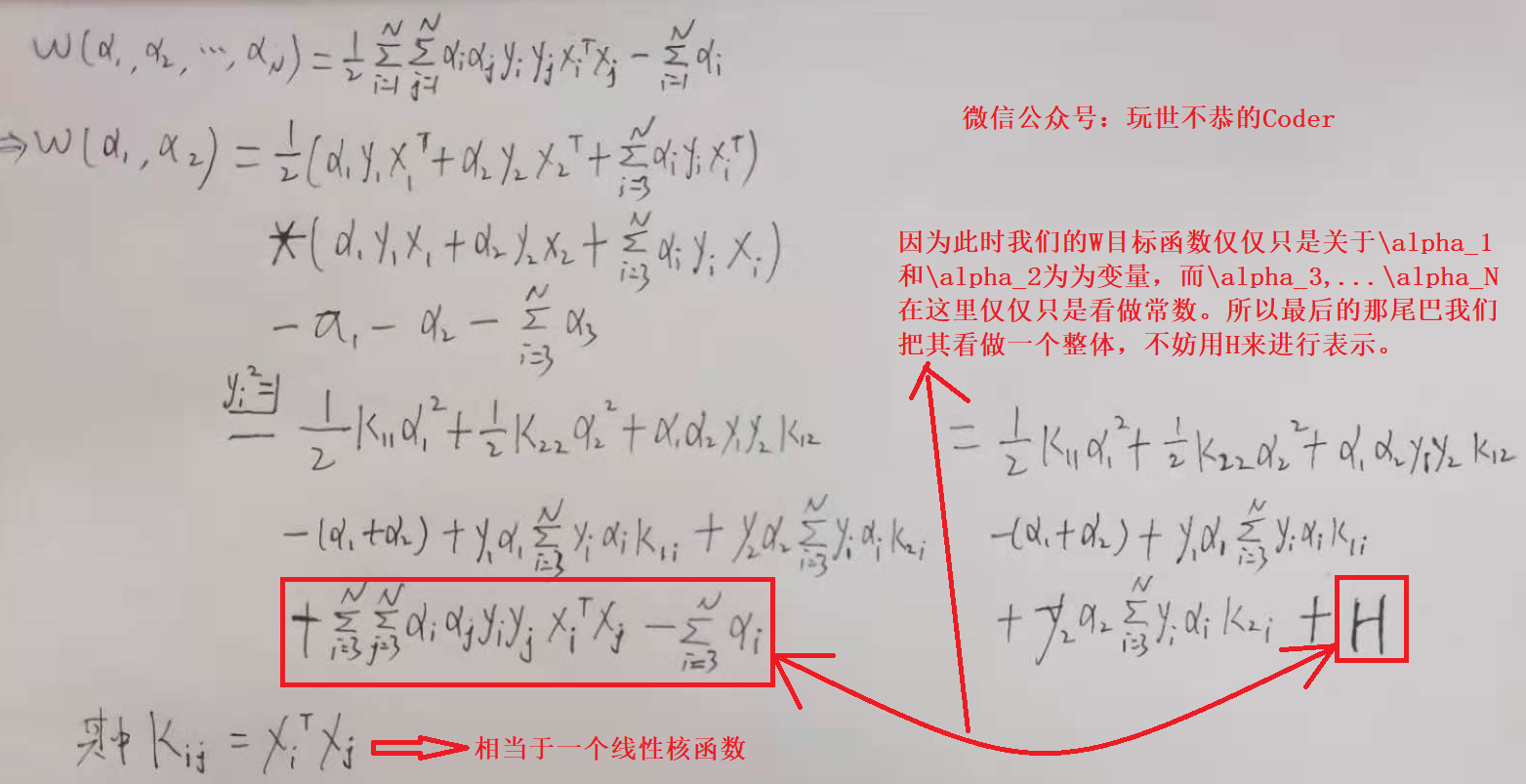W ( α 1 , α 2 ) = 1 2 K 11 α 1 2 + 1 2 K 22 α 2 2 + α 1 α 2 y 1 y 2 K 12 − ( α 1 + α 2 ) + y 1 α 1 ∑ i = 3 N y i α i K 1 i + y 2 α 2 ∑ i = 3 N y i α i K 2 i + H 其 中 K i j = x i T x j , H = ∑ i = 3 N ∑ j = 3 N α i α j y i y j x i T x j T − ∑ i = 3 N α i (4-6) \begin{aligned} W(\alpha_1, \alpha_2)= & \frac{1}{2}K_{11}\alpha_1^2+\frac{1}{2}K_{22}\alpha_2^2+\alpha_1\alpha_2y_1y_2K_{12} -(\alpha_1+\alpha_2) \\ & + y_1\alpha_1\sum_{i=3}^Ny_i\alpha_iK_{1i}+y_2\alpha_2\sum_{i=3}^Ny_i\alpha_iK_{2i} + H \\ & 其中K_{ij}=x_i^Tx_j,H=\sum_{i=3}^N\sum_{j=3}^N\alpha_i\alpha_jy_iy_jx_i^Tx_j^T-\sum_{i=3}^N\alpha_i \end{aligned} \tag{4-6}

∑ i = 1 N α i y i = 0 = > α 1 y 1 + α 2 y 2 = − ∑ i = 3 N α i y i = G = > α 1 = y 1 ( G − α 2 y 2 ) (4-7) \begin{aligned} & \qquad \sum_{i=1}^N\alpha_iy_i=0 \\ & => \alpha_1y_1+\alpha_2y_2=-\sum_{i=3}^N\alpha_iy_i=G \\ & => \alpha_1=y_1(G-\alpha_2y_2) \end{aligned} \tag{4-7}

W ( α 2 ) = 1 2 K 11 ( G − α 2 y 2 ) 2 + 1 2 K 22 α 2 2 + α 2 ( G − α 2 y 2 ) y 2 K 12 − [ y 1 ( G − α 2 y 2 ) + α 2 ] + ( G − α 2 y 2 ) ∑ i = 3 N y i α i K 1 i + y 2 α 2 ∑ i = 3 N y i α i K 2 i + H (4-8) \begin{aligned} W(\alpha_2)= & \frac{1}{2}K_{11}(G-\alpha_2y_2)^2+\frac{1}{2}K_{22}\alpha_2^2+\alpha_2(G-\alpha_2y_2)y_2K_{12}\\ & -[y_1(G-\alpha_2y_2) + \alpha_2]+(G-\alpha_2y_2)\sum_{i=3}^Ny_i\alpha_iK_{1i} \\ & + y_2\alpha_2\sum_{i=3}^Ny_i\alpha_iK_{2i}+H \end{aligned} \tag{4-8}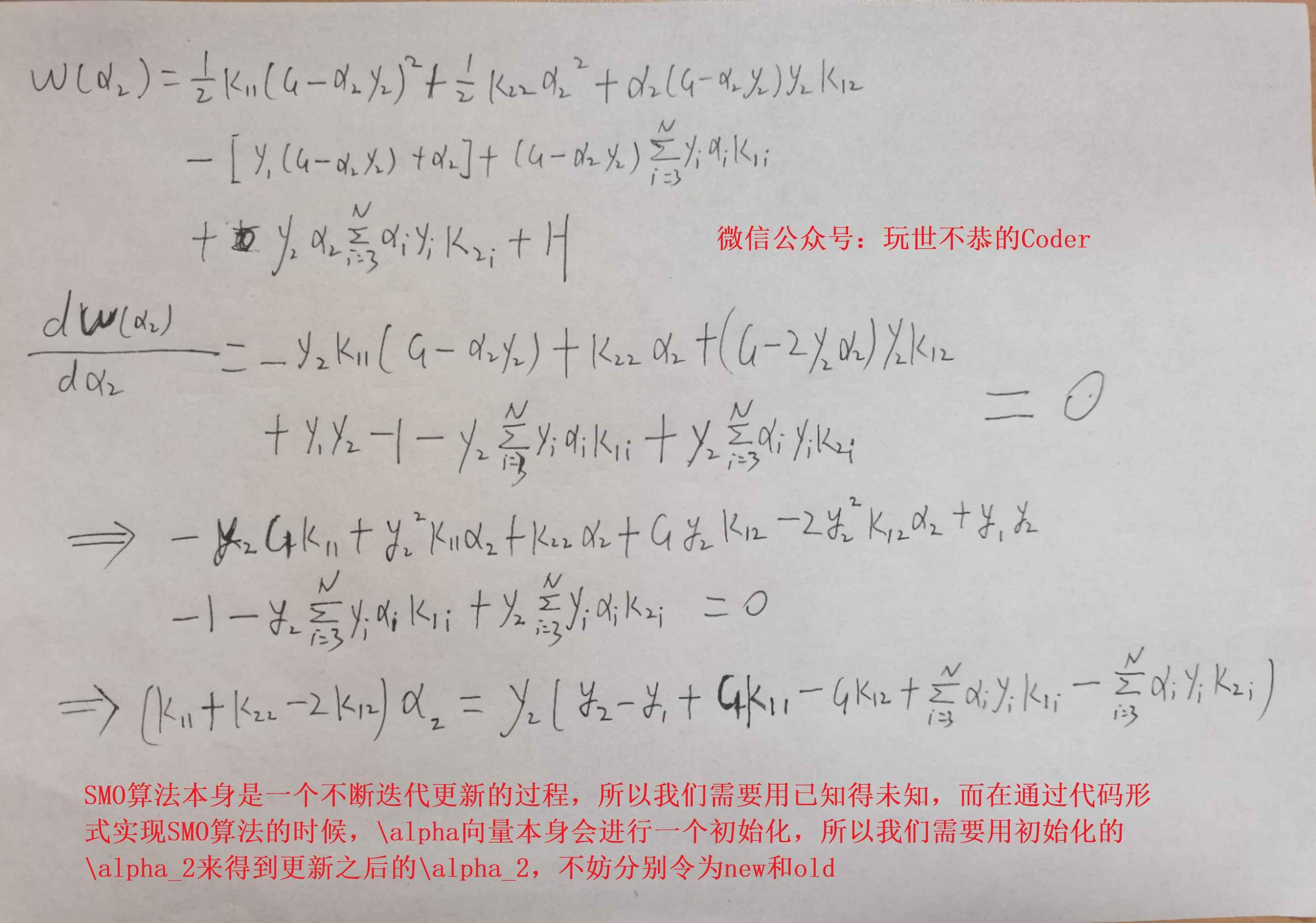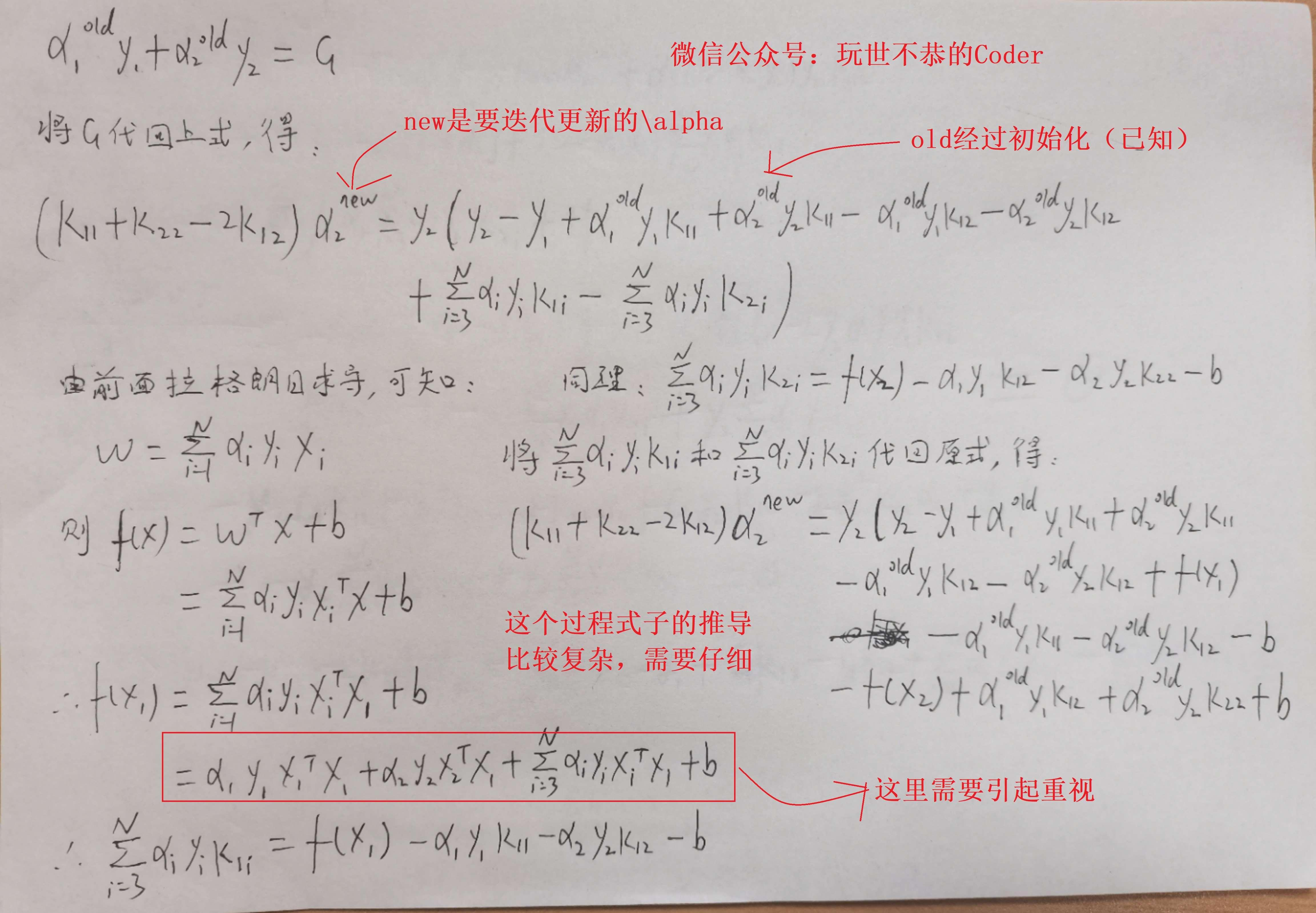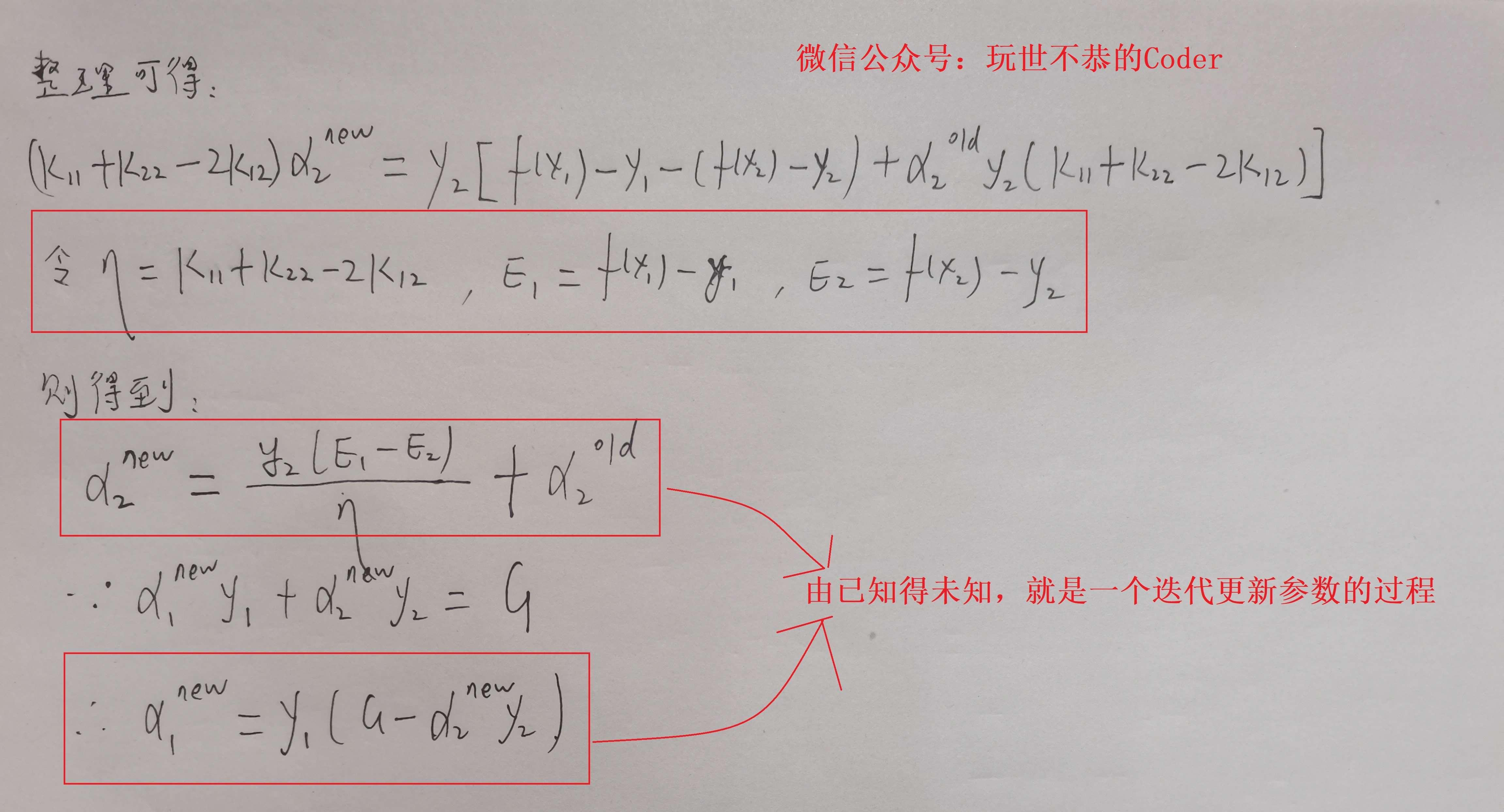( K 11 + K 22 − 2 K 12 ) α 2 = y 2 ( y 2 − y 1 + G K 11 − G K 12 + ∑ i = 3 N α i y i K 1 i − ∑ i = 3 N α i y i K 2 i ) (4-9) (K_{11}+K_{22}-2K_{12})\alpha_2=y_2(y_2-y_1+GK_{11}-GK_{12}+\sum_{i=3}^N\alpha_iy_iK_{1i}-\sum_{i=3}^N\alpha_iy_iK_{2i}) \tag{4-9}

α 1 o l d y 1 + α 2 o l d y 2 = G (4-9) \alpha_1^{old}y_1 + \alpha_2^{old}y_2=G \tag{4-9}

( K 11 + K 22 − 2 K 12 ) α 2 n e w = y 2 ( y 2 − y 1 + α 1 o l d y 1 K 11 + α 2 o l d y 2 K 11 − α 1 o l d y 1 K 12 − α 2 o l d y 2 K 12 + ∑ i = 3 N α i y i K 1 i − ∑ i = 3 N α i y i K 2 i ) (4-10) \begin{aligned} (K_{11}+K_{22}-2K_{12})\alpha_2^{new}= & y_2(y_2-y_1+\alpha_1^{old}y_1K_{11}+\alpha_2^{old}y_2K_{11} \\ & -\alpha_1^{old}y_1K_{12}-\alpha_2^{old}y_2K_{12} \\ & + \sum_{i=3}^N\alpha_iy_iK_{1i}-\sum_{i=3}^N\alpha_iy_iK_{2i}) \end{aligned} \tag{4-10}

α 2 n e w = y 2 ( E 1 − E 2 ) η + α 2 o l d α 2 n e w = y 1 ( G − α 2 n e w y 2 ) (4-11) \begin{aligned} & \alpha_2^{new}=\frac{y_2(E_1-E_2)}{\eta}+\alpha_2^{old} \\ & \alpha_2^{new}=y_1(G-\alpha_2^{new}y_2) \end{aligned} \tag{4-11}

PS：关于 η 、 E 1 、 E 2 \eta、E_1、E_2 是什么，请见上图中的内容。

w = ∑ i = 1 N α i y i x i w=\sum_{i=1}^N\alpha_iy_ix_i

0 ≤ α i ≤ C , i = 1 , 2 , 3 , . . . , N L ≤ α 2 n e w ≤ R (4-12) 0\leq\alpha_i\leq C ,\qquad i=1,2,3,...,N\\ L\leq\alpha_2^{new}\leq R \tag{4-12}

α 1 n e w y 1 + α 2 n e w y 2 = α 1 o l d y 1 + α 2 o l d y 2 = G (4-13) \alpha_1^{new}y_1+\alpha_2^{new}y_2=\alpha_1^{old}y_1+\alpha_2^{old}y_2=G \tag{4-13}

L = m a x ( 0 , α 2 o l d − α 1 o l d ) , R = m i n ( C , C + α 2 o l d − α 1 o l d ) y 1 ≠ y 2 L = m a x ( 0 , α 2 o l d + α 1 o l d − C ) , R = m i n ( C , α 2 o l d + α 1 o l d ) y 1 = y 2 (4-14) L=max(0, \alpha_2^{old}-\alpha_1^{old}),R=min(C,C+\alpha_2^{old}-\alpha_1^{old}) \quad y_1 \neq y_2\\ L=max(0, \alpha_2^{old}+\alpha_1^{old}-C),R=min(C,\alpha_2^{old}+\alpha_1^{old})\quad y_1=y_2 \tag{4-14}

y 1 = ∑ i = 1 N α i y i x i x 1 + b y_1=\sum_{i=1}^N\alpha_iy_ix_ix_1+b

b 1 n e w = y 1 − ∑ i = 3 N α i y i x i T x 1 − α 1 n e w y 1 x 1 T x 1 − α 2 n e w y 2 x 2 T x 1 (4-15) b_1^{new}=y_1-\sum_{i=3}^N\alpha_iy_ix_i^Tx_1-\alpha_1^{new}y_1x_1^Tx_1-\alpha_2^{new}y_2x_2^Tx_1 \tag{4-15}

b 1 n e w = b o l d − E 1 − y 1 ( α 1 n e w − α 1 o l d ) x 1 T x 1 − y 2 ( α 2 n e w − α 2 o l d ) x 2 T x 1 (4-16) b_1^{new}=b^{old}-E_1-y_1(\alpha_1^{new}-\alpha_1^{old})x_1^Tx_1-y_2(\alpha_2^{new}-\alpha_2^{old})x_2^Tx_1 \tag{4-16}

b 2 n e w = b o l d − E 2 − y 1 ( α 1 n e w − α 1 o l d ) x 1 T x 1 − y 2 ( α 2 n e w − α 2 o l d ) x 2 T x 2 (4-17) b_2^{new}=b^{old}-E_2-y_1(\alpha_1^{new}-\alpha_1^{old})x_1^Tx_1-y_2(\alpha_2^{new}-\alpha_2^{old})x_2^Tx_2 \tag{4-17}